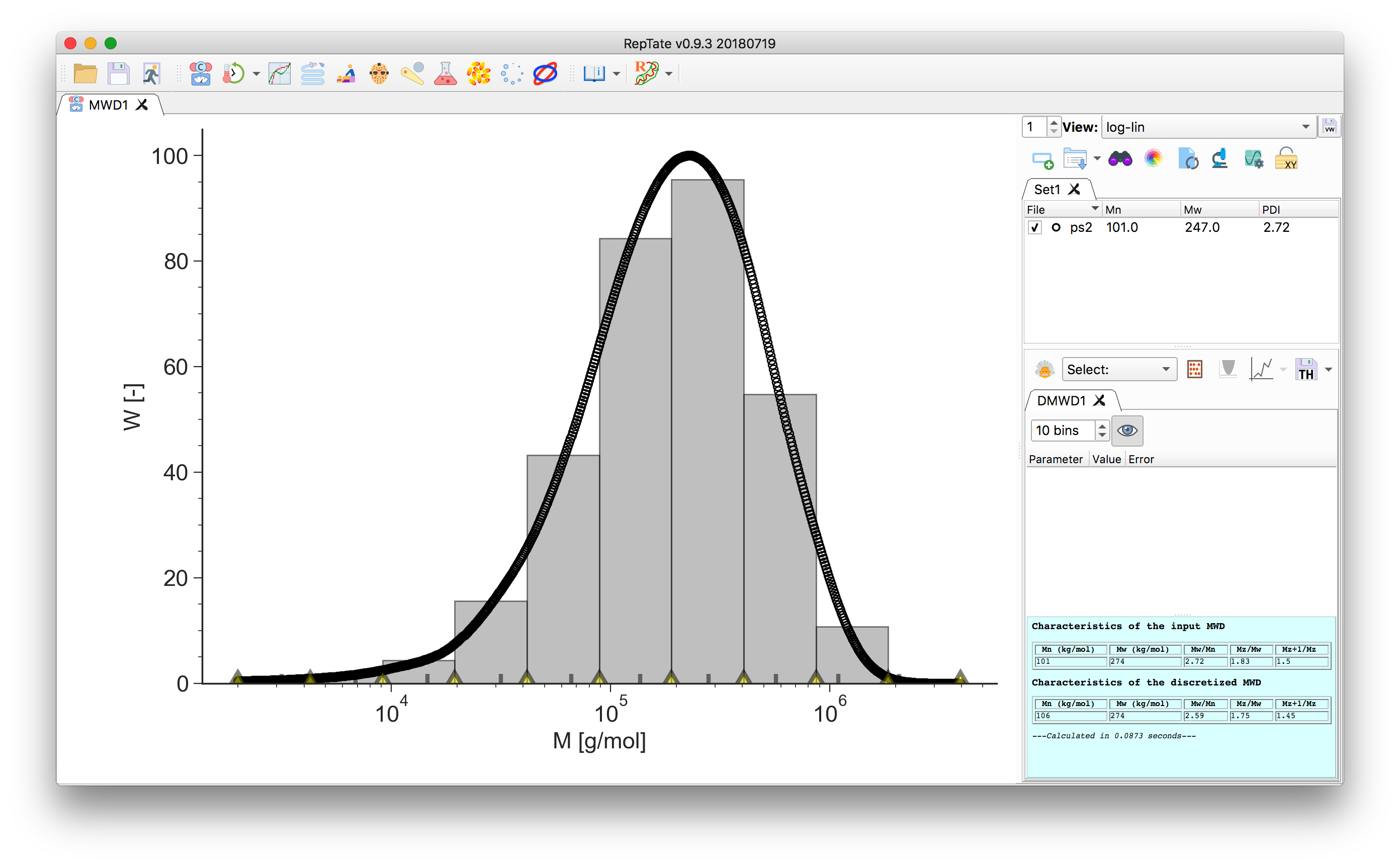# MWD: Theories¶

## MWD Discretization¶• The area, $$\phi_i$$, of each grey bin corresponds to the area under the data curve delimited by the bin edges. The height, $$h_i$$, of the bin is the area divided by the bin width (on a $$\log_{10}$$ scale).

You can change the number of bins and move the bin edges by dragging the yellow markers.

• In the bottom of the plot, the grey tick marks indicate the bin molecular weight taken as the weight-average molecular mass value across the bin width

$M_{w,i} = \frac{\sum w_j M_j}{\sum w_j}.$
1. To save the discretized molecular weight, click thebutton.

The output file contains a header with the moments $$M_n$$, $$M_w$$ and the PDI, and two columns. The first column is the molecular weight $$M_{w,i}$$ as indicated by the grey tick marks, the second column is $$\phi_i$$, the value of the area of the covered by the bin.

The sum of the areas should equal 1:

$\sum \phi_i = 1.$

## Log-Normal distribution¶

### Summary¶

Log-Normal distribution: the logarithm of the molecular weight is normally distributed

• Function
$W(M) = W_0 \frac{1}{\sqrt{2\pi\sigma^2}} \exp\left[ - \frac{\left(\ln{M}-(\ln{M_0} + \sigma^2)\right)^2}{2\sigma^2} \right]$
• Parameters
• logW0 $$\equiv\log_{10}(W_0)$$: Normalization constant.

• logM0 $$\equiv\log_{10}(M_0)$$

• sigma $$\equiv\sigma$$

## Generalized Exponential Function¶

### Summary¶

Generalized Exponential Function (GEX) for experimental molecular weight distributions.

• Function
$W(M) = W_0 \frac{b}{M_0 \Gamma\left(\frac{a+1}{b}\right)} \left(\frac{M}{M_0}\right)^{a} \exp\left[ -\left(\frac{M}{M_0}\right)^b \right]$
• Parameters
• logW0 $$\equiv\log_{10}(W_0)$$: Normalization constant.

• logM0 $$\equiv\log_{10}(M_0)$$: Proportional to $$M_n$$ and $$M_w$$.

• a : Parameter related to polydispersity and skewness

• b : Parameter related to polydispersity and skewness